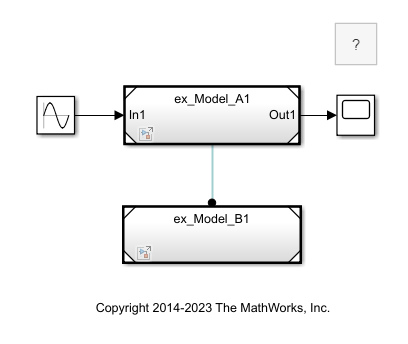## Simulink Function Blocks in Referenced Models

You can place Simulink Function blocks and function callers (such as Function Caller blocks and Stateflow® charts) in a referenced model, but doing so requires some special considerations:

• The referenced model must follow export-function model rules and be designated as an export-function model. See Export-Function Models Overview.

• Sometimes, you must explicitly define the argument data types for a Function Caller block.

These examples show four relationships between Function Caller blocks, Simulink Function blocks, and referenced models.

### Simulink Function Block in Referenced Model

In this example, the parent model contains a Function Caller block, and the referenced model, `Model_B`, contains a Simulink Function block. `Model_B` must follow export-function model rules.

The Function Caller block can determine the argument data types of the function. In the Function Caller block, you do not need to define the Input argument specification and Output argument specification parameters.

But since, by default, the Simulink Function block is scoped to the model, you must qualify a call to the function name with the Model block name.

To open a completed model, see `ex_referenced_model_with_simulink_function_block`.`Model_B` contains a Simulink Function block that defines a function for multiplying the input by 2. Because this model contains only a Simulink Function block, it satisfies export-function model rules. See Export-Function Models Overview.For `Model_B`, set the Configuration Parameters for the solver to satisfy export-function model rules:

• Type: `Fixed-step`.

• Solver: ```discrete (no continuous states)```.

#### Simulink Function Block in Referenced Model Placed in Subsystem

Once the Simulink Function block is identified as global by setting Function visibility to `global`, there are no limitations on where this referenced model can be placed.

For example, you could place `Model_B` with a Simulink Function block in a Subsystem block.### Function Caller Block in Referenced Model

In this example, the parent model contains a Simulink Function block, and a referenced model, `Model_A`, contains a Function Caller block. If you want to use this modeling pattering, the Function visibility parameter for the Trigger port block in the Simulink Function block must be set to `global`.

For the parent model, set the solver type to `Variable-step` or `Fixed-step`.

To open a completed model, see `ex_referenced_model_with_function_caller_block`.`Model_A` contains a Function Caller block.Since the Function Caller block cannot find the function in `Model_A`, you must set the Function visibility parameter for the Trigger block to `global` and specify the Function Caller block argument parameters:

• Input argument specification: Specify to match the Simulink Function block input argument data types, for example, `double(1.0)`.

Specify the argument specification for a Simulink Function block with the Data type parameter in the Input Argument and Output Argument blocks.

• Output argument specification: Specify to match the Simulink Function block output argument data types, for example, `double(1.0)`.

### Function and Function Caller Blocks in Separate Referenced Models

#### Function and Function Caller blocks in separate referenced models

This example shows a parent model containing two referenced models. Model_A is a referenced model with a Function Caller block. Model_B is a referenced model with a scoped Simulink Function block. Only Model_B with a Simulink Function block must follow export-function rules.#### Inside the referenced models

For `Model_A`, provide the argument specification as you do for the referenced model in Function Caller Block in Referenced Model. For `Model_B`, specify parameters as you do for the referenced model in Simulink Function Block in Referenced Model.

`Model_A` contains a Function Caller block. If the function is set to `global`, define the Input and Output Argument Specification parameters. If the function is set to `scoped`, provide the file name, not the block name, of the model where the function is expected to be resolved to as `y = Model_B.FunctionName(u)`.`Model_B` contains a Simulink Function block that defines a function for multiplying the input by 2. Because this model contains only a Simulink Function block, it satisfies export-function model rules. See Export-Function Models Overview.You can also use function ports to call a Simulink® function in a referenced model from another referenced model. See Call Simulink Functions in Other Models Using Function Ports.

### Function and Function Caller in the Same Model

In this example, the parent model contains one referenced model, `Model_C`, with both a Function Caller block and a scoped Simulink Function block.

• If the there is only one instance of `Model_C`, and the Configuration Parameter Total number of instances allowed per top model is set to `One`, the parent model simulates without error. Since `Model_C` does not export the function, it does not need to follow export-function rules.

• If the Configuration Parameter Total number of instances allowed per top model is set to `Multiple`, `Model_C` exports the function regardless if there is a single instance or multiple instances of the model. In both cases, Simulink displays an error. The model needs to follow export-function rules, but it does not because a Function Caller block is not allowed at the top-level of an export-function model.`Model_C` contains both a Function Caller block and a scoped Simulink Function block. If you want to use this modeling pattern, only one instance of `Model_C` is allowed in the parent model.